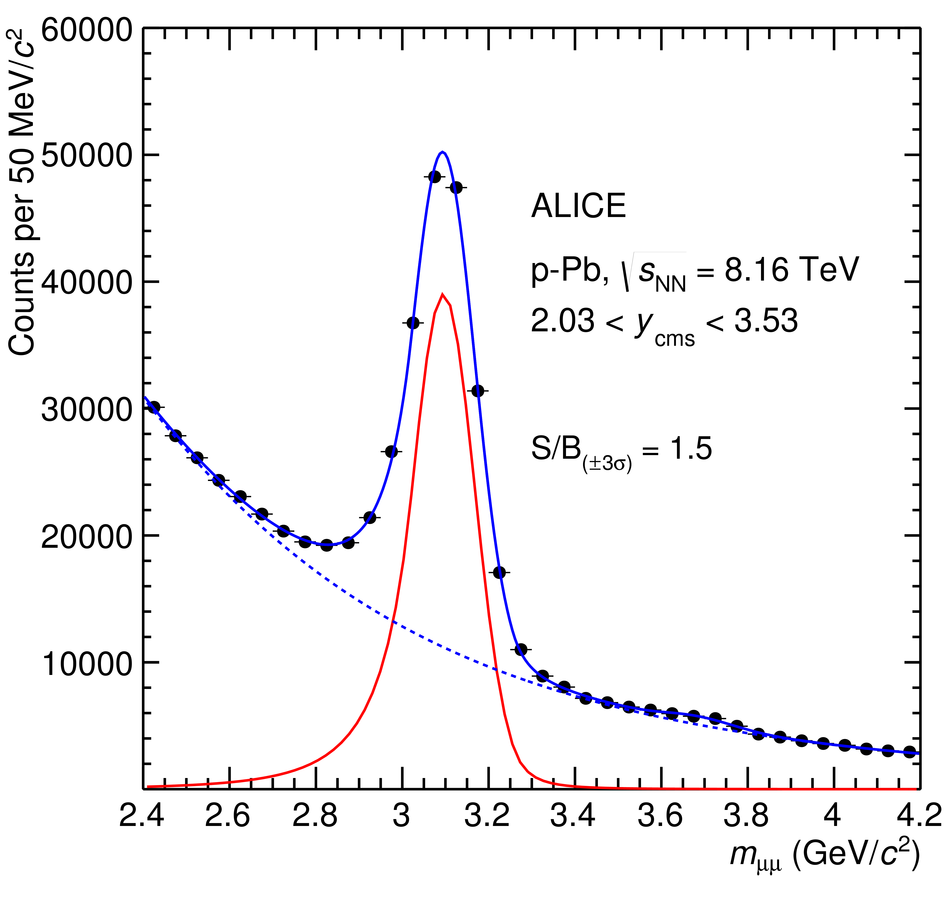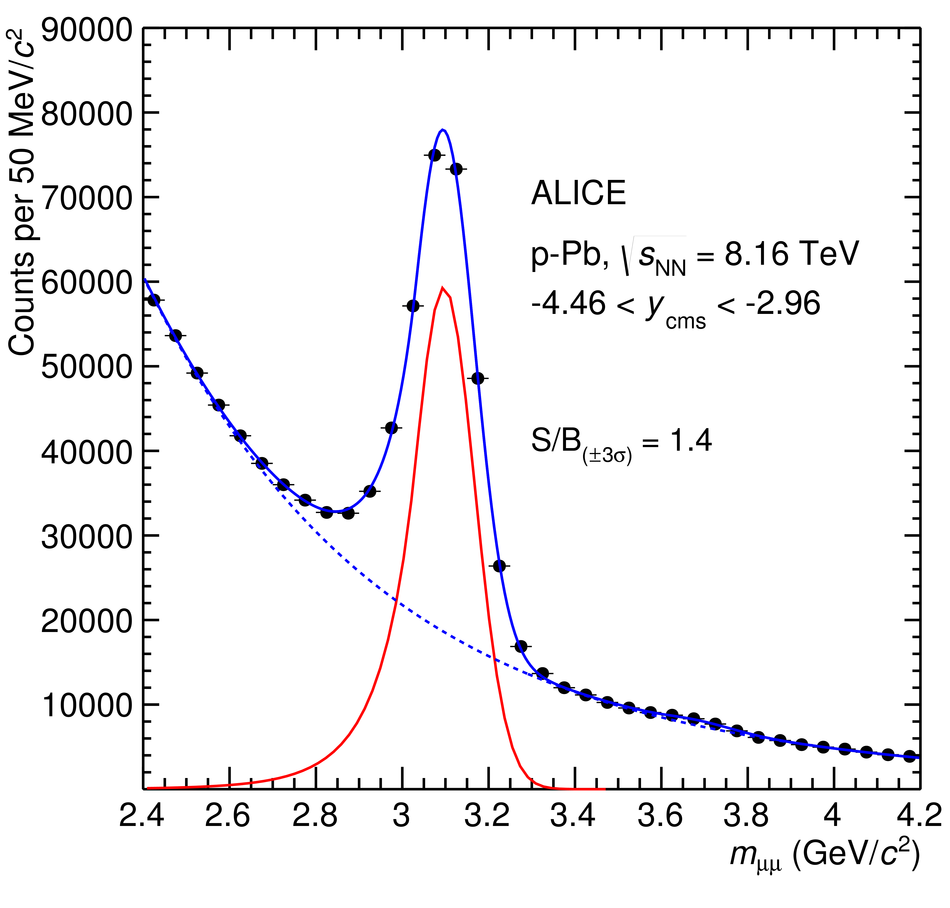# Figure 1

 Fits to the invariant mass distributions of opposite-sign dimuons with $p_{\rm T}< 20$ GeV/$c$. The left plot refers to $2.03< y_{\rm {cms}}< 3.53$, and that on the right to $-4.46< y_{\rm {cms}}< -2.96$. The shapes of both the resonances and the background are also shown.